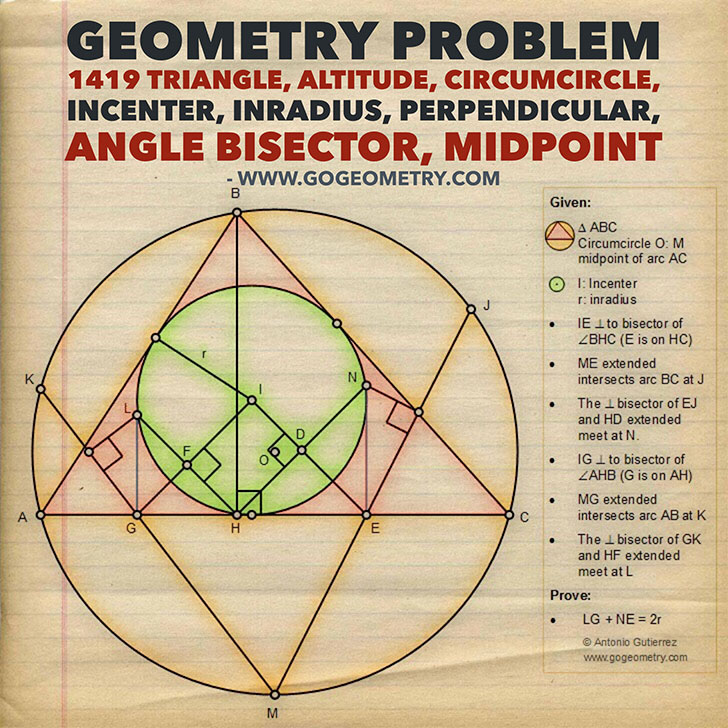Geometry Problem 1419: Triangle, Altitude, Circumcircle, Incenter, Inradius, Perpendicular, Angle Bisector, Midpoint

Proposition

The figure below shows a triangle ABC with the incenter I, inradius r, the altitude BH, the circumcircle O and the midpoint M of arc AC. A line segment through I and perpendicular to the bisector of the angle BHC at D intersects HC at E. ME extended intersects arc BC at J. HD extended and the perpendicular bisector of EJ meet at N. A line segment through I and perpendicular to the bisector of the angle AHB at F intersects AH at G. MG extended intersects arc AC at K. HF extended and the perpendicular bisector of GK meet at L. Prove that LG + NE = 2r.Geometric Art of Problem 1419: Sketching, Typography, iPad Apps